Courses

# Ideal Gas Mixtures and Liquid Solutions Civil Engineering (CE) Notes | EduRev

## Civil Engineering (CE) : Ideal Gas Mixtures and Liquid Solutions Civil Engineering (CE) Notes | EduRev

The document Ideal Gas Mixtures and Liquid Solutions Civil Engineering (CE) Notes | EduRev is a part of the Civil Engineering (CE) Course Thermodynamics.
All you need of Civil Engineering (CE) at this link: Civil Engineering (CE)

Ideal Gas Mixtures and Liquid Solutions

We next explore the development of a quantitative definition of the chemical potential in terms of the volumetric properties and composition of mixtures. We have observed earlier that just as ideal gas state is a reference for real gas properties, ideal gas mixtures play the same role with respect to real gas mixtures. Therefore, it is instructive to establish the property relations for ideal gas mixture first. Consider the constitution of an ideal gas mixture (containing N species) at a given temperature (T) and pressure (P). To obtain n moles of the total mixture we need to bring together ni moles of each species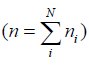at temperature T but at a pressure pi which corresponds to the partial pressure that each species would exert in the final mixture. If Vt is the total volume of the mixture, the following set of relations hold.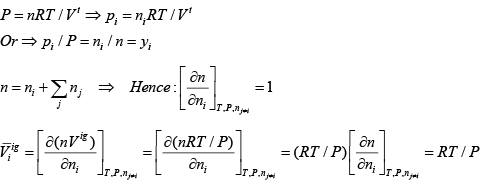......(6.53)

But the molar volume of the ith species  Vig = RT/P

Hence it follows: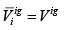......(6.54)

The last result indicates that the molar volume for a species does not change between its pure state and in an ideal gas mixture at the same T & P.  It may then be concluded that for an ideal gas mixture the properties of each species are independent of that of the other ones. This may be easy to appreciate as the concept of an ideal gas is premised on the idea that the intermolecular interaction is non-existent in such a state. This conclusion leads to the well-known Gibbs theorem:

“Except for volume all other partial molar property of a species in an ideal-gas mixture is equal to the corresponding molar property of the species as a pure ideal gas at a temperature same as that of the mixture, but at a pressure equal to its partial pressure in the mixture.”

In mathematical terms :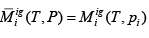......(6.55)

As an example let us consider the case of enthalpy of an ideal gas mixture. By Gibbs theorem: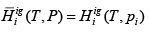......(6.56)

But, as the enthalpy of an ideal gas is independent of pressure it follows that: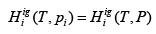......(6.57)

It follows: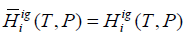......(6.58)

By the standard definition, the enthalpy of the mixture is: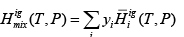......(6.59)
Thus, using eqns. 6.56 – 6.59 we get: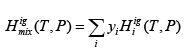......(6.60)

It follows: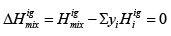......(6.61)

Employing the same reasoning: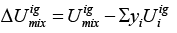......(6.62)

The molar entropy of mixing of ideal gas mixture, however, is not zero. As stated above, the formation of 1 mole of mixture results from bringing together yi moles each species at T and partial pressure pi to form a mixture at T and P, Hence for isothermal mixing, yi moles of each species goes from (T, pi) to (T, P). Therefore: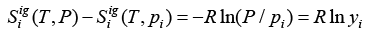......(6.63)

On transposing: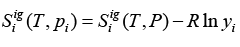......(6.64)

But: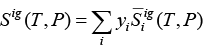......(6.65

So: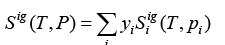......(6.66)

Using eqns. 6.63 - 6.67, one obtains: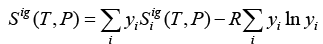......(6.67)

On applying the partial molar property operation (as given by eqn. 6.4) on 6.58, it may be shown that: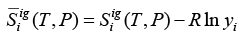......(6.68)

It further follows: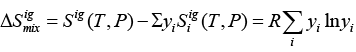......(6.69)

For Gibbs free energy relation we start from: G = H − TS

For an ideal gas mixture: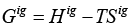......(6.70)

Taking the partial molar property derivative: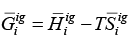......(6.71)

On putting eqns. 6.58 and 6.59 into 6.71 we get the following relation for the chemical potential of each species in an ideal gas mixture: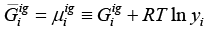......(6.72)

Using eqns. 6.51 and 6.58, it may be also shown that: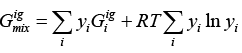......(6.73)

The Ideal Solution:

We have already seen that owing to the fact that pure ideal gases and mixtures are not subject to intermolecular interactions the partial molar properties (apart from volume) of each species is the same as that of the pure species at the same temperature and pressure. In other words each species “sees” no difference in their environment in pure or mixed state. One can conceptually extend this idea to posit an ideal solution behaviour which may serve as a model to which real-solution behavior can be compared. Consider a solution of two liquids, say A and B. If the intermolecular interaction in the pure species, (i.e., A-A and B-B) is equal to the cross-species interaction A-B, neither A nor B type molecules will “see” any difference in their environment before and after mixing. This is in a sense the same condition as one obtains with idea gas mixtures. Hence an identical set of ideal solution property relations may be constructed based on the model of ideal gas mixture. By convention while describing properties of liquid solutions mole fractions yi are replaced by xi. The following relations therefore, derive for ideal (liquid) solution properties (denoted by a superscript ‘id’):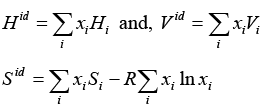......(6.74 & 6.75)

Hence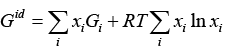......(6.76)

Lastly,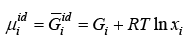......(6.77)

As we will see later the ideal solution model can also serve to describe the behaviour of mixtures of real gases or solids.

Offer running on EduRev: Apply code STAYHOME200 to get INR 200 off on our premium plan EduRev Infinity!

## Thermodynamics

56 videos|92 docs|33 tests

,

,

,

,

,

,

,

,

,

,

,

,

,

,

,

,

,

,

,

,

,

;